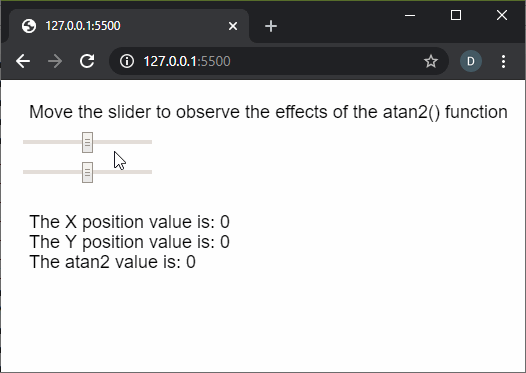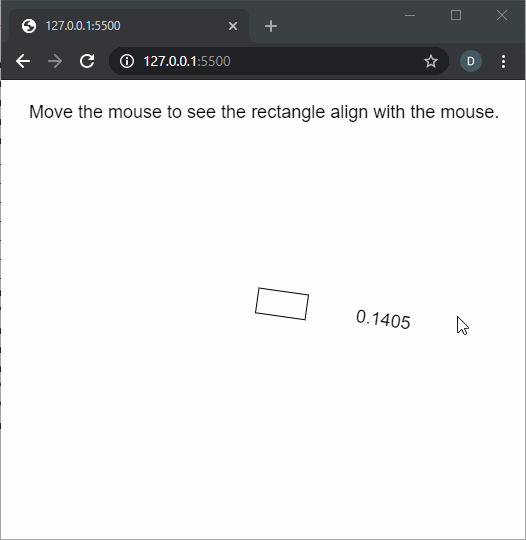# p5.js | atan2() Function

The atan2() function in p5.js is used to calculate the angle from a specified point of origin measured from the positive x-axis. The values are returned in the range of PI to -PI as floating points. It is commonly used for orienting geometry to the position of the cursor.

Syntax:

`atan2(y, x)`

Parameters: This function accepts two parameters as mentioned above and described below.

• y: It is a number that specifies the y-coordinate of the point.
• x: It is a number that specifies the x-coordinate of the point.

Return Value: It returns a number that denotes the arc tangent of the given point.

Below examples illustrate the atan2() function in p5.js:

Example 1:

 `function` `setup() { ` `  ``createCanvas(500, 200); ` `  ``textSize(18); ` ` `  `  ``text(``"Move the slider to observe the effects"` `         ``+ ``" of the atan2() function"``, 20, 30); ` ` `  `  ``sliderXPos = createSlider(-200, 200, 0, 1); ` `  ``sliderXPos.position(20, 50); ` ` `  `  ``sliderYPos = createSlider(-200, 200, 0, 1); ` `  ``sliderYPos.position(20, 80); ` `} ` ` `  `function` `draw() { ` `  ``clear(); ` `  ``text(``"Move the slider to observe the effects"` `         ``+ ``" of the atan2() function"``, 20, 30); ` ` `  `  ``sliderXVal = sliderXPos.value(); ` `  ``sliderYVal = sliderYPos.value(); ` ` `  `  ``atan2Val = atan2(sliderXVal, sliderYVal); ` ` `  `  ``text(``"The X position value is: "` `+ sliderXVal, 20, 140); ` `  ``text(``"The Y position value is: "` `+ sliderYVal, 20, 160); ` `  ``text(``"The atan2 value is: "` `+ atan2Val, 20, 180); ` `} `

Output:Example 2:

 `function` `setup() { ` `  ``createCanvas(500, 400); ` `  ``textSize(18); ` `  `  `  ``text(``"Move the mouse to see the rectangle"` `        ``+ ``" align to it."``, 20, 30); ` `} ` `  `  `function` `draw() { ` `  ``clear(); ` `  ``text(``"Move the mouse to see the rectangle"` `        ``+ ``" align with the mouse."``, 20, 30); ` `  `  `  ``// Move the rectangle to the ` `  ``// middle of the screen ` `  ``translate(width / 2, height / 2); ` `  `  `  ``// Use the atan2() function to find ` `  ``// the value according to the mouse ` `  ``// coordinates ` `  ``let adjustedValue = atan2(mouseY - height / 2, ` `                            ``mouseX - width / 2); ` `  ``rotate(adjustedValue); ` `  `  `  ``// Draw a rectangle ` `  ``rect(0, 0, 50, 25); ` `  `  `  ``text(adjustedValue.toFixed(4), 100, 20); ` `} `

Output:Online editor: https://editor.p5js.org/

Reference: https://p5js.org/reference/#/p5/atan2My Personal Notes arrow_drop_upCheck out this Author's contributed articles.

If you like GeeksforGeeks and would like to contribute, you can also write an article using contribute.geeksforgeeks.org or mail your article to contribute@geeksforgeeks.org. See your article appearing on the GeeksforGeeks main page and help other Geeks.

Please Improve this article if you find anything incorrect by clicking on the "Improve Article" button below.

Article Tags :

Be the First to upvote.

Please write to us at contribute@geeksforgeeks.org to report any issue with the above content.